# Angular Momentum Practice Problems

An error occurred trying to load this video.

Try refreshing the page, or contact customer support.

Coming up next: The Laws of Thermodynamics

### You're on a roll. Keep up the good work!

Replay
Your next lesson will play in 10 seconds
• 0:02 Angular Momentum:…
• 0:51 Sample Moments of Inertia
• 1:23 Point Mass Problems
• 4:34 Rotating Cylinder Problems
• 5:55 Lesson Summary

Want to watch this again later?

Timeline
Autoplay
Autoplay
Speed

#### Recommended Lessons and Courses for You

Lesson Transcript
Instructor: Erin Monagan

Erin has been writing and editing for several years and has a master's degree in fiction writing.

If you need some practice on problems involving angular momentum, then this is the place you need to be! In this lesson, we'll work on conservation of momentum, rotating bodies and moments of inertia.

## Angular Momentum: Basic Equation

In linear momentum we use the equation P = mv, where P is the momentum, m is the mass in kilograms, and v is the velocity in meters per second. The angular momentum equivalent is: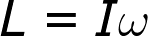Where L is angular momentum, I is the moment of inertia, and omega is the angular velocity. The angular velocity can be related to the linear velocity, v, if you know the radius, r, from the center of rotation by using the equation w = v/r. However, the moment of inertia for any object is determined by three factors: its mass, shape, and axis of rotation.

## Sample Moments of Inertia

The moment of inertia I of a point mass moving in a circle of radius r: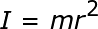The moment of inertia of a disc: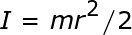The moment of inertia of a thin rod about its center: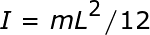The moment of inertia of a thin rod about its end: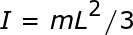## Point Mass Problems

The easiest types of angular momentum problems are those that involve a point mass, or point particle rotating around a center of an axis. Examples of point mass problems can be anything from a ball on a string to planetary sized bodies. Using the linear momentum equation, and substituting in the moment of inertia of a rotating point mass, we get L = mrv. So, these problems depend on mass, radius, and linear velocity.

#### Problem 1

What is the angular momentum around the catcher of a baseball thrown at 40 m/s? The weight of the baseball is 145 kilograms and on a wild pitch the catcher has extended his arm 1.25 m from his center of rotation.

L = mrv

L = (.145 kg)(1.25 m)(40 m/s)

L = 7.25 kg/m2/s

#### Problem 2

A ball is rotating on a string 5ft from the end of a hollow pipe with a linear velocity of 10 ft/s. The string continues down the pipe to the other end. What would the new linear velocity of the ball be if you pulled the string 3 ft, thereby shortening the turning radius of the ball?

Since linear momentum is conserved between the two states (as long as we ignore friction, the weight of the string and the diameter of the pipe) the new angular momentum and old angular momentum are going to be equal.

L(old) = L(new)

mrv(old) = mrv(new)

m(5)(10) = m(5-3)(v)

The masses cancel out, leaving:

50 = 2v

v = 25 ft/s

To unlock this lesson you must be a Study.com Member.

### Register to view this lesson

Are you a student or a teacher?

### Unlock Your Education

#### See for yourself why 30 million people use Study.com

##### Become a Study.com member and start learning now.
Back
What teachers are saying about Study.com

### Earning College Credit

Did you know… We have over 160 college courses that prepare you to earn credit by exam that is accepted by over 1,500 colleges and universities. You can test out of the first two years of college and save thousands off your degree. Anyone can earn credit-by-exam regardless of age or education level.